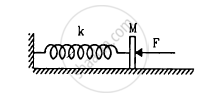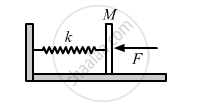Department of Pre-University Education, KarnatakaPUC Karnataka Science Class 11
Advertisement Remove all ads

# In Figure Following K = 100 N M−1, M = 1 Kg and F = 10 N. (A) Find the Compression of the Spring in the Equilibrium Position. (B) a Sharp Blow by Some External Agent Imparts a Spe - Physics

Sum

In following figure k = 100 N/m M = 1 kg and F = 10 N. (a) Find the compression of the spring in the equilibrium position. (b) A sharp blow by some external agent imparts a speed of 2 m/s to the block towards left. Find the sum of the potential energy of the spring and the kinetic energy of the block at this instant. (c) Find the time period of the resulting simple harmonic motion. (d) Find the amplitude. (e) Write the potential energy of the spring when the block is at the left extreme. (f) Write the potential energy of the spring when the block is at the right extreme.
The answer of (b), (e) and (f) are different. Explain why this does not violate the principle of conservation of energy.Advertisement Remove all ads

#### Solution

It is given that :
Spring constant, k = 100 N/m,
Mass of the block, M = 1 kg
Force, F = 10 N(a) In the equilibrium position,

$F = kx$

where x is the compression of the spring, and
k is the spring constant.

$\therefore x = \frac{F}{k} = \frac{10}{100}$

$= 0 . 1 m = 10 cm$

(b) The blow imparts a speed of 2 ms-1 to the block, towards left.
Potential energy of spring, U =$\frac{1}{2}k x^2$

Kinetic energy,

$K$=$\frac{1}{2}M v^2$

(c) Time period $\left( T \right)$  is given by,

$T = 2\pi\sqrt{\frac{M}{k}}$

$= 2\pi\sqrt{\frac{1}{100}} = \frac{\pi}{5} s$

(d) Let A be the amplitude.
Amplitude is the distance between the mean and the extreme position.
At the extreme position, compression of the spring will be (A + x).

As the total energy in S.H.M.  remains constant, we can write:

$\frac{1}{2}k \left( A + x \right)^2 = \frac{1}{2}k x^2 + \frac{1}{2}M v^2 + Fx$

$= 2 . 5 + 10x$

$\therefore$ 50(A + 0.1)2 = 2.5 + 10x

$\Rightarrow$ 50A2 + 0.5 + 10A = 2.5 + 10A

$\Rightarrow$ 50A2 = 2

$\Rightarrow A^2 = \frac{2}{50} = \frac{4}{100}$

$\Rightarrow A = \frac{2}{10} m = 0 . 2 m = 20 cm$

(e) Potential Energy at the left extreme will be,

$P . E . = \frac{1}{2}k \left( A + x \right)^2$

$= \frac{1}{2} \times 100 \times \left( 0 . 1 + 0 . 2 \right)^2$

$= 50 \times \left( 0 . 09 \right) = 4 . 5 J$

(f) Potential Energy at the right extreme is calculated as:
Distance between the two extremes = 2A

$P . E . = \frac{1}{2}k \left( A + x \right)^2 - F\left( 2A \right)$

= 4.5 − 10 (0.4) = 0.5 J

As the work is done by the external force of 10 N, different values of options (b), (e) and (f) do not violate the law of conservation of energy.

Is there an error in this question or solution?
Advertisement Remove all ads

#### APPEARS IN

HC Verma Class 11, 12 Concepts of Physics 1
Chapter 12 Simple Harmonics Motion
Q 16 | Page 253
Advertisement Remove all ads
Advertisement Remove all ads
Share
Notifications

View all notifications

Forgot password?